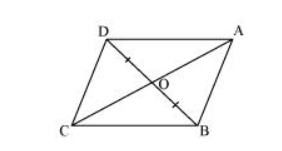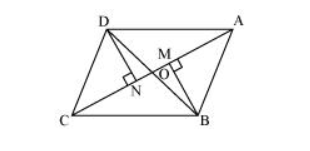# In the given figure, diagonals AC and BD of quadrilateral ABCD intersect at O such that OB = OD.

Question.

In the given figure, diagonals AC and BD of quadrilateral ABCD intersect at O such that OB = OD. If AB = CD, then show that:

(i) $\operatorname{ar}(D O C)=\operatorname{ar}(A O B)$

(ii) $\operatorname{ar}(\mathrm{DCB})=\operatorname{ar}(\mathrm{ACB})$

(iii) DA II CB or ABCD is a parallelogram.Solution:Let us draw $D N \perp A C$ and $B M \perp A C$.

(i) In $\triangle \mathrm{DON}$ and $\triangle \mathrm{BOM}$,

$\angle D N O=\angle B M O$ (By construction)

$\angle D O N=\angle B O M$ (Vertically opposite angles)

$O D=O B$ (Given)

By AAS congruence rule,

$\Delta \mathrm{DON} \cong \triangle \mathrm{BOM}$

$\therefore D N=B M \ldots(1)$

We know that congruent triangles have equal areas.

$\therefore$ Area $(\Delta D O N)=$ Area $(\Delta B O M) \ldots(2)$

In $\triangle D N C$ and $\triangle B M A$,

$\angle D N C=\angle B M A$ (By construction)

$C D=A B$ (Given)

$D N=B M$ [Using equation (1)]

$\therefore \Delta \mathrm{DNC} \cong \Delta \mathrm{BMA}(\mathrm{RHS}$ congruence rule)

$\Rightarrow \operatorname{Area}(\Delta \mathrm{DNC})=\operatorname{Area}(\Delta \mathrm{BMA}) \ldots(3)$

On adding equations $(2)$ and $(3)$, we obtain

Area $(\Delta D O N)+$ Area $(\Delta D N C)=\operatorname{Area}(\Delta B O M)+\operatorname{Area}(\Delta B M A)$

Therefore, Area $(\Delta D O C)=$ Area $(\triangle A O B)$

(ii) We obtained,

Area $(\Delta D O C)=$ Area $(\triangle A O B)$

$\Rightarrow$ Area $(\Delta D O C)+$ Area $(\Delta O C B)=\operatorname{Area}(\Delta A O B)+\operatorname{Area}(\Delta O C B)$

(Adding Area ( $\triangle \mathrm{OCB}$ ) to both sides)

$\Rightarrow$ Area $(\triangle D C B)=$ Area $(\triangle A C B)$

(iii) We obtained,

Area $(\triangle \mathrm{DCB})=$ Area $(\triangle \mathrm{ACB})$

If two triangles have the same base and equal areas, then these will lie between the same parallels.

$\therefore$ DA $\| C B \ldots(4)$

In $\triangle \mathrm{DOA}$ and $\triangle \mathrm{BOC}$,

$\angle D O A=\angle B O C$ (Vertically opposite angles)

$O D=O B($ Given $)$

$\angle O D A=\angle O B C$ (Alternate opposite angles)

By ASA congruence rule,

$\triangle \mathrm{DOA} \cong \triangle \mathrm{BOC}$

$\therefore D A=B C \ldots(5)$

In quadrilateral $A B C D$, one pair of opposite sides is equal and parallel $(A D=B C)$

Therefore, $A B C D$ is a parallelogram.